Browse by Topic
Related Topics

# A “Trivial” Probability Problem

I am a junkie for a good math problem. Over the weekend, I encountered such a good problem on a favorite subject of mine–probability. It’s the last problem from the article “A Mathematical Trivium” by V. I. Arnol’d, Russian Mathematical Surveys 46(1), 1991, 271–278.

It’s short enough to reproduce in its entirety: “Find the mathematical expectation of the area of the projection of a cube with edge of length 1 onto a plane with an isotropically distributed random direction of projection.” In other words, what is the average area of a cube’s shadow over all possible orientations?

This blog post explores the use of Mathematica to understand and ultimately solve the problem. It recreates how I approached the problem.

### Projection of a square on a line

Before tackling the case of the cuboid, I started with a unit square, randomly rotated around its center of mass, with the intent to find the average length of its projection on a horizontal axis.

For the sake of simplicity, I placed the center of the mass of the square at the origin.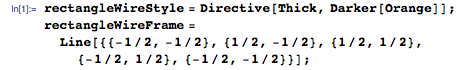I found the left and right boundaries of the projection as the smallest and largest x coordinates of the vertices of the square, rotated around the origin through an angle of α degrees: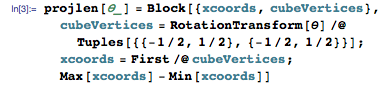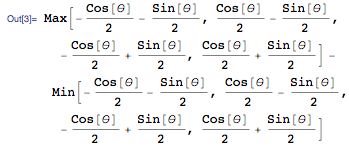I then combined these functions within the Manipulate to be able to dynamically change the angle of rotation: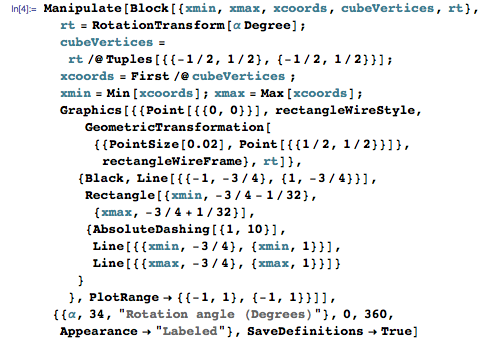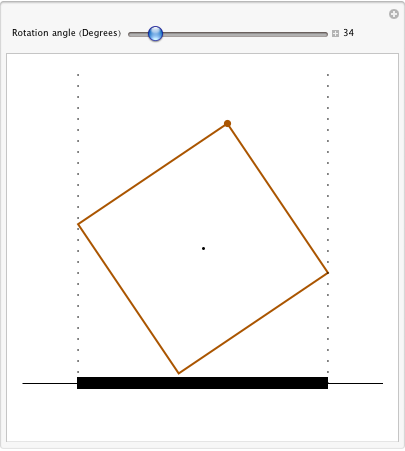Here’s the plot of the length of the projection as the function of the rotation angle: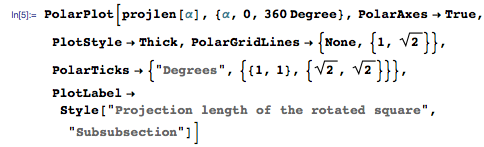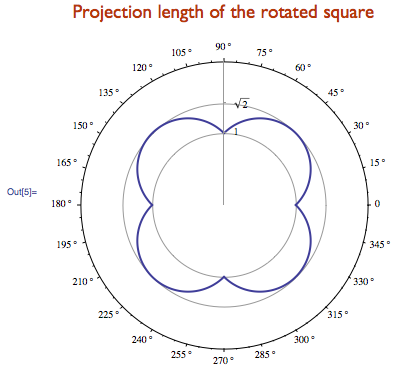Assuming the rotation angle α is uniformly distributed in the interval 0 ≤ α < 360, I readily found the expected length of the projection, that is, the average length of the square’s shadow: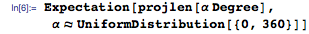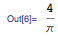### Change the frame of reference of the rectangle

That was easy enough, but in order to take the victory to 3D, I needed to change the point of view. Instead of rotating the square, I rotated the line we project on.

Perspective of the wire frame: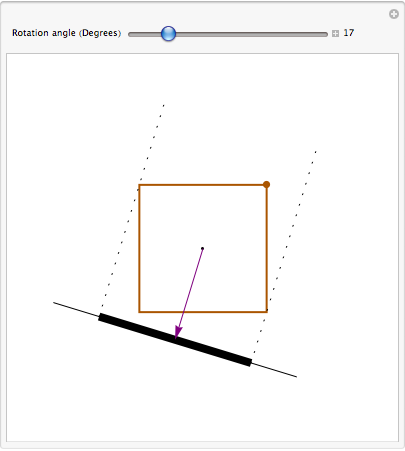I found this change of perspective illuminating, as it made me think that the length of the projection is the sum of the lengths of the projections of the two sides of the square visible from the plane.

The length of the projection of a segment of length &#8467 with unit normal vector n1 onto a line with unit normal vector n2 equals &#8467 Dot[n1,n2].

The projection of each side of the square only contributes if Dot[n1,n2] is positive (i.e. the side is visible); otherwise it is hidden behind other sides. The length of the shadow is thus the sum of the contributions of the east, west, top, and bottom sides of the square: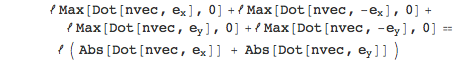Thus the length of the projection is the sum of the absolute values of coordinate components of the normal vector nvec. I implemented this way of computing the length of the shadow in a function: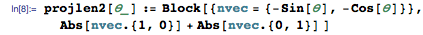And, of course, it agrees with the earlier way of computing the projection length: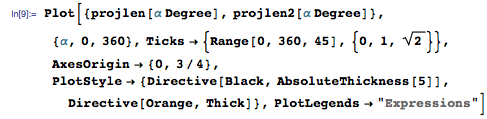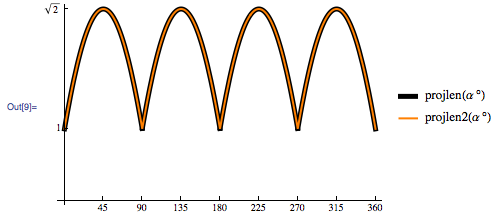Naturally, the expectation is the same: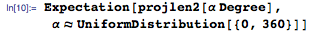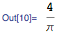### The case of the cube

Guided by the insight gained by considering the square, I adopted the reference frame of the cuboid, whose center of mass is situated at the origin. The cuboid casts its shadow on a plane whose orientation is parametrized by its perpendicular (i.e. normal) vector nvec.

I started by straightforwardly building projections of each face of the cuboid and drawing them together. First I defined this helper function to project a vector xvec onto a plane with normal nvec: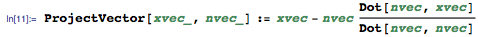The following function gives vertex coordinates of a face in the (i,j) plane with the other coordinate being z0. Here i and j range over {1,2,3}, which designates the x-, y-, and z– directions, and z0 ranges over {-1/2,1/2}: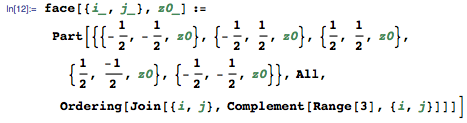I then defined a function to project each face onto a plane with normal vector nvec and produce the corresponding 3D polygon: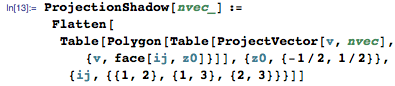The area of a polygon that happens to be a quadrangle projected onto a plane with normal nvec is computed as the sum of the areas of two triangles that the quadrangle is split into by a diagonal: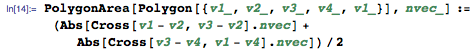With these utilities in place, I was ready to visualize the projection of the cuboid with edge of length one, whose center of mass is situated at the origin and whose sides are aligned with the coordinate axis. I parametrized the unit normal vector to the projection plane using spherical coordinates θ and φ: {sin(θ) sin(φ),sin(θ) cos(φ),cos(θ)}.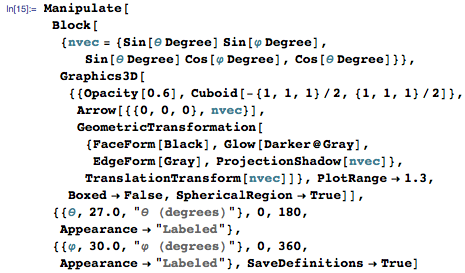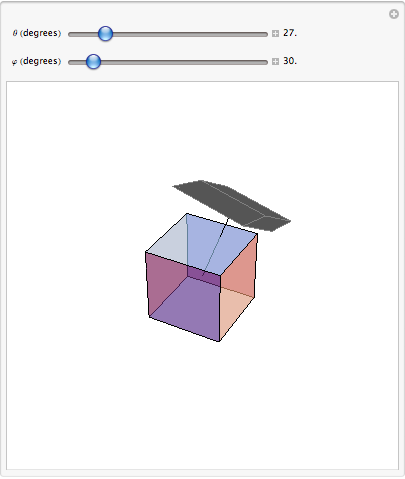Since at any one time, only three of the cuboid’s faces are visible, and since the area contributed by the invisible faces is the same (just imagine a parallel plane on another side of the cuboid), I sum over all the six faces and divide the result by two. For a particular orientation nvec=={-1,2,3}√14 of the plane, the area of the projection therefore is computed as follows: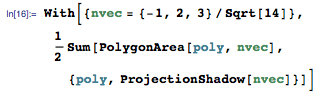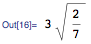Applying the insight learned from solving the 2D case, I checked if the area of the projection was again the sum of the absolute values of dot products of the normal vector nvec with axis vectors: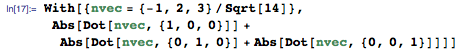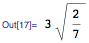Bingo! This makes sense, I thought. Each term corresponds to the area of one of at most three visible faces. Indeed, consider a patch of area S on a plane with unit normal vector n1. The area of the projection of the patch onto another plane with unit normal vector n2 equals S Abs[Dot[n1,n2]].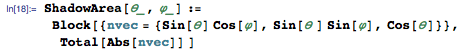Here is the spherical plot of the area of the shadow cast by the cuboid onto a plane with unit normal vector {sin(θ) sin(φ),sin(θ) cos(φ),cos(θ)} as the function of Euler’s angles θ and φ: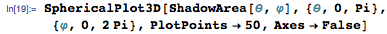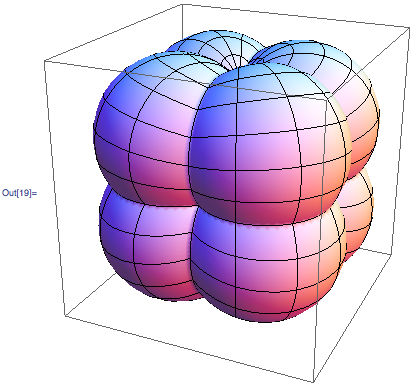The minimum of the area function corresponds to the area of one face, which equals 1, and the maximum of √3 corresponds to the projection on the plane, whose normal vector aligns with the cuboid’s diagonal: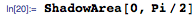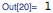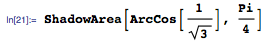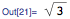I was then almost ready to tackle the expected value of the area. For the normal unit vector {nx,ny,nz}, the expected projection area is: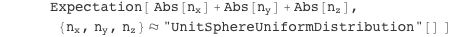The surface area of an infinitesimal patch (θ,θ+ ⅆθ)×(φ,φ+ⅆφ) of the unit sphere is well known to be sin(θ) ⅆθφ. Dividing the infinitesimal area by the total surface area of the unit sphere, I obtain the infinitesimal probability measure, corresponding to the uniform distribution on the unit sphere: ⅆ S(θ,φ)==1/(4 π)sin(θ) ⅆθφ.

And, finally, the expected projection area equals: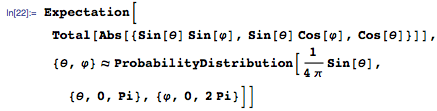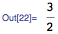Of course, I could simplify the computation, using the symmetry argument: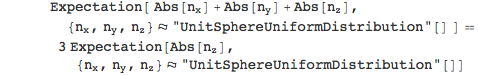It is a well-known fact (also see this relevant question on math.stackexchange.com) that each individual component of a random vector, uniformly distributed on the unit sphere, follows a uniform distribution on the interval (-1,1). With this insight, the answer can be worked out in one’s head, explaining why this problem was deemed by Arnol’d a “trivium”: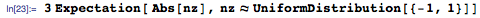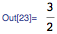### Going to higher dimensions

The insight I gained allowed me to easily construct the answer for the case of the d-dimensional hypercube, projected on the randomly oriented hyperplane: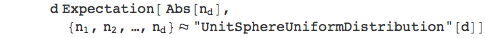I simply needed to know the distribution of a component of the d-dimensional random unit vector.

The computations are simple, and are based on hyperspherical coordinates (e.g. see Wikipedia: n-sphere). The infinitesimal hyperspherical area also factors as (Sin[θ1]d-2θ1)(Sin[θ2]d-3θ2)⋯(Sin[θd-2]ⅆθd-2)ⅆθd-1. Since the nd==cos(θ1), I needed to find the normalization constant for the quasi-density Sin[θ1]d-2: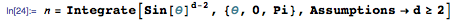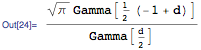Therefore the expected area of the projection of d-hypercube is: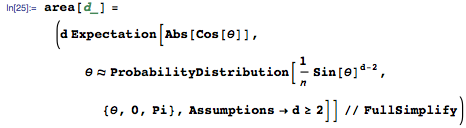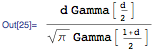Alternatively, I could use the closed-form result for the probability density function (PDF) of the distribution from here: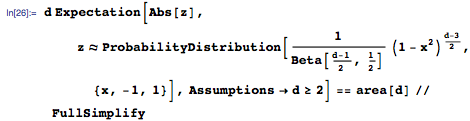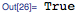I concluded this rewarding exploration by reproducing the results obtained earlier and tabulating results for higher dimensions: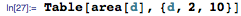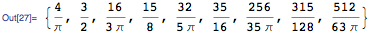The average area of the shadow grows boundlessly with space dimension, as the number of faces that contribute to its area also increases: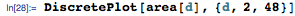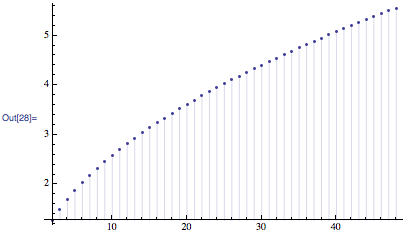In the high-dimensional space, the area scales as a square root of the dimension d: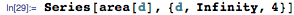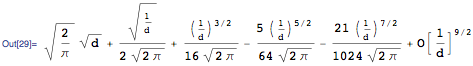This concluded my exploration of Arnol’d’s trivium problem with Mathematica. The use of Mathematica led to many “Aha!” moments, and enabled me to readily answer various “What if…” questions. It is my hope that I was able to convey the excitement of the discovery process largely accelerated with the use of Mathematica, and to inspire you to begin explorations of your own.